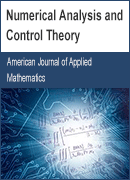Coming Special IssueExpiring Date:
Jul. 10, 2020
share
Special Issues
Expand the Popularity of Your Conference
Publish conference papers as a Special Issue
Send your Special Issue proposal to:
review_specialissue@sciencepg.com
Submit Hot TopicsSubmit
Home / Journals / American Journal of Applied Mathematics / Numerical Analysis and Control Theory
Numerical Analysis and Control Theory

Shahed University, Tehran, IranIntroduction
My major interests in the mentioned special issues are categorized into two parts. First, providing new efficient numerical methods for the numerical treatment of fractional delay differential equations which have many applications in a wide range of science and engineering. Second, Analysis and control of delay systems. Recently, I pay a lot of time for the numerical solution of stochastic differential equations.

Aims and Scope:

1. Numerical solution of delay differential equations
2. Numerical solution of fractional differential equations
3. Numerical solution of fractional delay differential equations
4. Stability analysis of delay differential equations
5. Stabilization by the delayed feedback method
6. Numerical solution of stochastic differential equations (recently)
PUBLICATION SERVICES